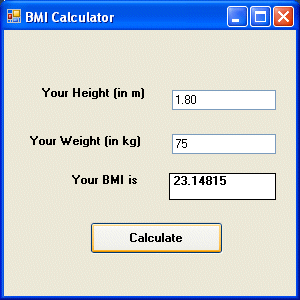# Visual Basic 2013 Lesson 11: Managing Mathematical Operations

#### [Lesson 10] << [Contents] >> [Lesson 12]

Basically, mathematical operations form an integral part of computer programming. Indeed, we can write Visual Basic 2013 code to instruct the computer to perform mathematical calculations. Furthermore, we can create VB applications that are able to solve complex mathematical problems.

For example, we can develop VB applications like simultaneous equations Solver and Factors Finder. Besides that, we can use mathematical operations to create programs like the slot machine, star war, amortization calculators and so on. In order to write a VB program that involves mathematical operations, we need to use the standard arithmetic operators. In addition, we must also use some mathematical formulas as well as equations.

The Visual Basic 2013 mathematical operators are very similar to the normal arithmetic operators, only with some slight variations. The list of VB 2013 mathematical operators are shown in table 11.1 below:

#### Table 11.1: Mathematical Operators

Operator Mathematical Function Example
Subtraction  10-4=6
^  Exponential  3^2=9
*  Multiplication  5*6=30
/  Division  21/7=3
Mod  Modulus(returns the remainder of an integer division)  15 Mod 4=3
\  Integer Division(discards the decimal places)  19/4=4

#### Example 11.1

In this example, we insert two text boxes, four labels, and a button. Now, click the button and enter the code as shown below. When you run the program, it will perform four basic arithmetic operations and displays the results on the four labels.

```Private Sub Button1_Click(ByVal sender As System.Object, ByVal e As System.EventArgs) Handles Button1.Click
Dim num1, num2, difference, product, quotient As Single
num1 = TextBox1.Text
num2 = TextBox2.Text
sum=num1+num2
difference=num1-num2
product = num1 * num2
quotient=num1/num2
Label1.Text=sum
Label2.Text=difference
Label3.Text = product
Label4.Text = quotient
End Sub```

#### Example 11.2

This program employs the Pythagoras Theorem to calculate the length of hypotenuse c given the length of the adjacent side a and the opposite side b. For those of  you who have forgotten the formula for the Pythagoras Theorem, it is written as

```c^2=a^2+b^2
```

#### The Code

```Private Sub Button1_Click(ByVal sender As System.Object, ByVal e As System.EventArgs) Handles Button1.Click
Dim a, b, c As Single
a = TextBox1.Text
b = TextBox2.Text
c=(a^2+b^2)^(1/2)
Label3.Text=c
End Sub
```

#### Example 11.3: BMI Calculator

In today’s world, many people are obese and it could affect their health seriously. In fact, obesity has proven by the medical experts to be one of the main factors that bring many adverse medical problems, including the cardiovascular disease. Based on scientific research, if your BMI is more than 30, you are considered obese. You can refer to the following range of BMI values for your weight status.

Underweight = <18.5
Normal weight = 18.5-24.9
Overweight = 25-29.9
Obesity = BMI of 30 or greater

To calculate your BMI, it is not necessary to consult a doctor, you can simply use a calculator or a DIY computer program instead. In this example, we will show you how to create a BMI calculator. The BMI value can be calculated using the formula weight/( height )^2, where weight is measured in kg and height in meter. If you only know your weight and height in lb and feet, then you need to convert them to the metric system.

```Private Sub Button1_Click(ByVal sender As System.Object, ByVal e As System.EventArsgs) Handles Button1.Click
Dim height, weight, bmi As Single
height = TextBox1.Text
weight = TextBox2.Text
bmi = (weight) / (height ^ 2)
Label4.Text = bmi
End Sub
```

The output is shown in the Figure 11.1 below. In this example, your height is 1.80m( about 5 foot 11),your weight is 75 kg( about 168Ib), and your BMI is about 23.14815. The reading suggests that you are healthy. (Note; 1 foot=0.3048, 1 lb=.45359237 kilogram)From the above examples, you can see that writing visual basic 2013 code that involves arithmetic operations is relatively easy. Here are more projects you work on:

Area of a triangle
Area of a rectangle
Area of a circle
Volume of a cylinder
Volume of a cone
Volume of a sphere
Compound interest
Future value
Mean
Variance
Sum of angles in polygons
Conversion of lb to kg
Conversion of Fahrenheit to Celsius